History Research
Volume 3, Issue 3, May 2015, Pages: 41-47

Mathematics Hundred Years Before and Now

Eka Ratna Acharya

Central Department of Education, Mathematics Education, Tribhuvan University, Kirtipur, Kathmandu, Nepal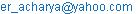Eka Ratna Acharya. Mathematics Hundred Years Before and Now. History Research. Vol. 3, No. 3, 2015, pp. 41-47. doi: 10.11648/j.history.20150303.11

Abstract: Conservation of manuscript and using history of mathematics is the Goal of this paper. It is based on fundamental unifying concept of classical and modern mathematics. The actual differences in classical and modern mathematics are paradigm. In early mathematics there is no variation in branches but modern mathematics is divided into different branches. Geometry and Calculus are modified in context of applications and process. In practice we recognized and accepted old as gold, so this paper tries to link up classical to modern approach to change the paradigm in mathematics with focusing the evidences of mathematical developments.

Keywords: Conservation, Modern Mathematics, Classical Mathematics, Paradigm Shift

1. Introduction

I'm trying to think about a paradigm shift in mathematics with comparing mathematics before 100 years and now on the basis of contents. A Paradigm shift is a major change from one way of thinking to another way of thinking in mathematics. Since awareness is prerequisite to all acceptable changes of theory and Science. The awareness helps to paradigm shift in mathematics. Change of paradigm occurs if the old design runs out of intellectual stream in context of existing mathematics.

People think that New mathematics never overturns old mathematics and they think that they don’t get paradigm shift in mathematics. But mathematics based in nature and practice that are always changeable. Thus the mathematics is obviously shifts in accordance of science and technology with arts. A few things come to mind as paradigm shifts in mathematics basically views in mathematics. Late 19th century, the invention and use of symbolic logic helps to shift the mathematics in conceptual base. In 19th - 20th century is the time of rise of abstract algebra, which combined and generalized large areas of existing mathematics into a more cohesive whole. It also gave birth too many of the major fields of modern advanced mathematics like algebraic geometry, algebraic number theory, the rise of analysis. An important part of mathematics, which is calculus of derivative, was used by Bhaskaracharya in 12th century. In 17th century Newton and Leibnitz made calculus rigorous. The shifting in mathematics is the changing between classical and modern concept of using and solving strategies of mathematics.

The Early 20th century is the fall of the Italian school of algebraic geometry. This is more of a local paradigm shift. Mid of the 19th century there is rise of hyperbolic/non - Euclidean geometry and the fall of the parallel postulate. Early 20th century is the foundational crisis in mathematics because there was not new development in mathematics but there was rigorous review in mathematics. This review helps to linkage to old mathematics to new mathematics.

In every aspect we accept that old is gold. So we should linkup between classical and modern mathematics. We lack but open eye and ear to find the orient’s marvels here through the comparison between old and new mathematics. Paradigm shifting in mathematics is the basis of shifting of development. But our different levels mathematics is only rote learning. There is no modification, creativity and historical basis. This is due to the lack up historical linkage in mathematics. The following slide expressed the classical approach of mathematics which is very basis of the daily used mathematics.

In modern concept the above prosodic mathematical statement can be explain as, O marvelous girl! Out of a group of swans, 7/2 times the square root of the number is playing on the shore of a tank. The two remaining ones are playing with amorous fight, in the water. What is the total number of swans? The solution of this problem can be obtain as, let total number of swans = x then by question, (7/2) √x +2= x. That is, 4x2 – 64x +16 = 0, provide x =16 and ¼ but ¼ is impossible then required number of swans =16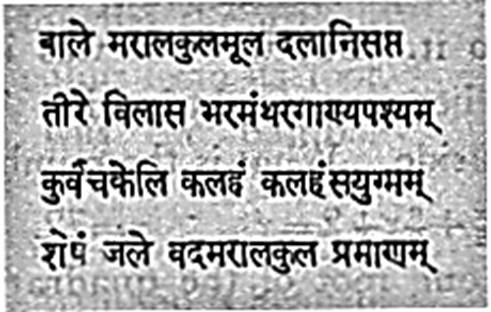Figure 1. A poetic (prosody) form of a practical example of an equation.

2. Evidences of Old Manuscripts

Let us share few evidences of mathematics before 100 years. The few old mathematical documents are expressed here for example: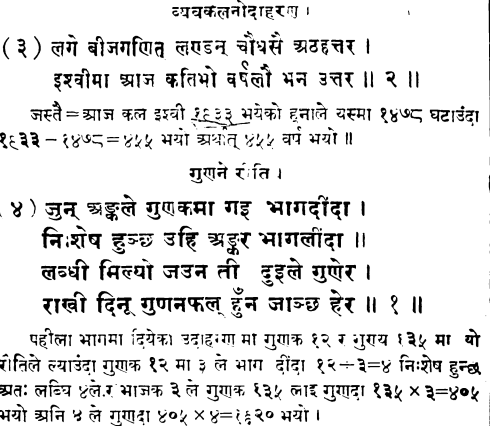Figure 2. A poetic expression for addition & multiplication.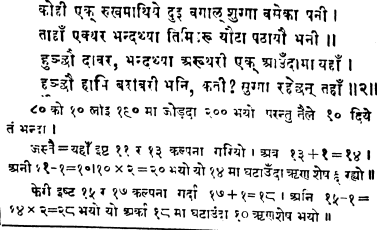Figure 3. A prosody expression of the simultaneous linear equations.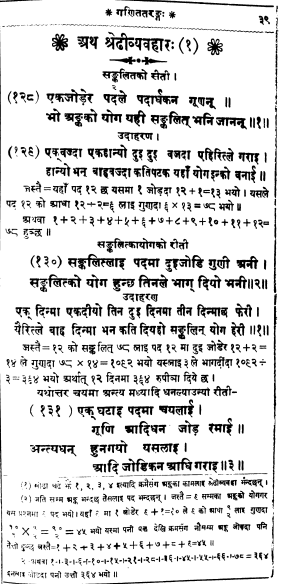Figure 4. The poetic expression for finding arithmetic sum of the numbers.

This problems can be solved by converting these prosody style of mathematical equation into symbolic form, like as: Let x and y be the number of parrots in first and second group then as described in prosody we have, x+1=2 (y - 1) or x - 2y= - 3…… (i) and x - 1=y+1 or x - y=2… (ii) solving (i) and (ii) we get x=7 and y =5. Hence 7 & 5 are the number of parrots. Such types of problems are found now so in comparison with before and now there is not seen more changes the verbal problems.

In above figure the first statement explains the formula for the sum of the numbers which are in arithmetic series. According to this statement the sum of the first ‘n’ natural numbers can be calculated by the formula, where ‘n’ is the number of terms. This formula can be used as, S = {2a+ (n - 1) d} equivalently write as,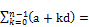{2a+ (n - 1) d}

For truth of the above expression can be illustrated; if k = 0 then first term is ‘a’; if k = 1 then the second term is a +d; if k = 2 then third term is a +2d similarly if k= 3 then fourth term is a +3d and their arithmetic sum is arrange like as ‘a+a+d+a+2d+a+ (4 - 1)d = 4a +6d = 2{2a+ (4 - 1)d}=  {2a+ (4 - 1)d}.

But these formulae are in verbal form in that expression. By using this formula an example is described here. The next statement explained the formula for the sum of the numbers as in the series 1+3+6+10+15+21+…………+78; the sum of this series can be find by using the formula, Where ‘l’ is the last term; n, the number of term, that is the division of 3 to the multiple of last term to the sum of the number of term with 2. The third statement states the rule as the formula, . Thus these types of popular formulae are used in verbal way.

Similarly other examples of mathematical developments that consider as link to classical to modern by differed the method. Here that is the rule of three for calculating cube root which differed to Bhaskaracharya view was given by Gopal Pande in his book Vyaktachandrika.It is described as below with an example of calculation of cube root of ‘123456789’.The following steps to be adopted by Gopal Pande for the calculation of cube roots of the given number.

1)    Mark the points above the digits at last on right then leave two digits toward left and put points each time. Such as the first point is marked above 9, second above 6 and third above 3 as shown,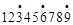2)    Count the number of points marked above the digits which gives the number of digits of the cube roots with leaving the digits for decimal place of the cube roots. Here the number of digits in the cube root is 3, since there are 3 points.

3)    Assume that the number bears the first point from left (or last point from the right) as a single number and subtract the possible cube of a number from it. Here the first point from left bears up to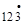, so we should subtract the cube of 4 that is 64 and take down the digits up to 2nd point and note that 4 is the first digit from the left of the cube root.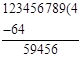4)    From (3) we get 59456 then we should multiply 59456 by ten and divide it by the number as the multiple of 1000 in the relation of the difference of cube of (first digit of cube root +1) and the cube of the first digit of the cube root. Here this two numbers are 53 and 43 and their differences as explained above is125 – 64 = 61.

5)    Then from (4) we have,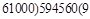Then we get 2nd digit of the cube root i.e. 9. Thus the number is 49. Again processed as (4) we get,

503 - 493=7351 and 453=117649

6)    Again subtract cube of 49 from 123456789 and take down the digit up to 3rd point as follow;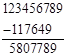7)    Divided by 7351000 to (5807789×10) as mentioned above, i.e.,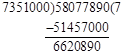8)    (497)3=122763473 then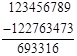Hence digits of cube root before decimal place are 497.This completes the digits of cube roots before decimal place. Next for after decimal place we should introduce three zeros to the remainder obtained in above and proceed as (4) and (5). It is note that the difference of cube mentioned as 4 can be obtained as.

(498)3 - (497)3=3{(497)2+497} +1=742519

9)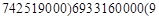Thus the cube root of 123456789=497.9 

The following figures explain the rule of calculation of cube root. For evidences they are shown as picture.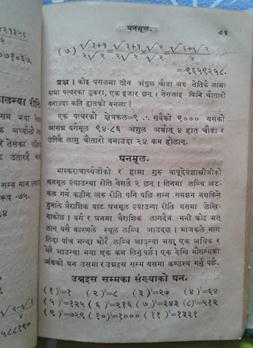Figure 5. A page of the book Vyaktachandrika of Gopal Pande & application of rule of three in cube root.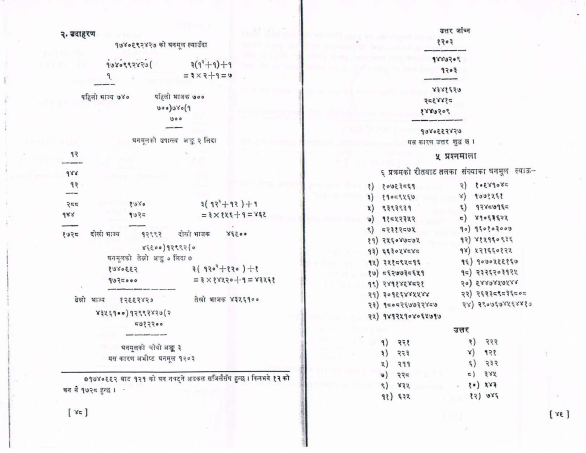Figure 6. Naya Raj Pant’s explanation of rule of three for cube root as Gopal Pande.

Let us move in calculus which is known as the advanced mathematics in modern mathematics. The verbal statements derived from Lilavati of Bhaskaracharya as, Tatkaliki gatiha syat tatha krite jata gatiha = n y - 1,this gives the derivative of the function. These define the process of first order derivative in Bhaskaracharya Lilavati. This shows the findings of derivatives in 12th century A. D 

So the derivative that is the calculus was developed nearly 5th century A.D ago than Newtown and Leibnitz. Now days derivative is introduce in our course in plus two levels but before 9th century ago it was introduced high school level course. In the basis of these evidences there are not considerable changes in the content rather than language and pedagogical aspect. These representative evidences are directing to investigate the how our contents, algorithm and problem solving style differ than previous 100 years old mathematics.

The mathematics based in Vedic period was highly advanced and very short, so it was easy to understanding . But now days it is saying ‘elaborated & reasoning’; in spite of these stills it is based on rote learning and lengthy due to this our learner cannot assimilate easily.

Here is the question; how much our learning paradigm shift in content base, algorithm base and problem solving style? Even we are saying our mathematics is changed. In comparison with content, algorithm and problem solving style cannot see changes with assimilating the learners understanding. So linking with historical base & follow up some rules then we can be upgrade our levels of mathematics in content wise, algorithm wise and problem solving style. For this some evidences are as follows.

2.1. Application of Derivatives to Solve Quadratic Equations

Formula: The comparison of first order derivative of the given quadratic expression to the positive and negative square root of the discriminate of that expression. That is, the first order derivative of the expression = ±√ (discriminate of the quadratic equation) .

Example: Consider the equation 5x2 - 3x - 2 = 0

Now, applying the above formula then we get, 10x - 3 = ± √ (- 3)2 - 4.5. (- 2) ± 7 x =1 or

2.2. Derivatives and Turning Points

If f (x) = g (x)/h (x) then the formula: f (x) = g’ (x)/h’ (x)

Example: f (x) =  = g (x)/h (x) [let]

f (x) = g’ (x)/h’ (x) =

0r f (x) =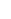= x = ±.

Therefore f (x) = at x =1 and f (x) = -  at x= - 1. Hence turning points are

(1, ) and (- 1, - )

2.3. Derivatives and Factorization

The following relation gives the formula quadratic equations.

First order derivative = sum of the factors Example: x2 +3x+2

The factors are (x+1) and (x+2); derivatives of the expression is, f’ (x) = 2x+3.

Therefore f’ (x) = (x+1) + (x+2) = ΣA, where ΣA is the sum of the factors.

2.4. Formula for Finding the Factors Cubic Expression with the Help of Derivative and Vice Versa

Formula f’ (x) = ΣA.B, where ΣA.B is the sum of the all pair of the factors.

Example: factorize the expression x3+6x2+11x+6

Therefore f’ (x) = (x+1) (x+2) + (x+1) (x+3) + (x+2) (x+3) =ΣA.B

That is f’ (x) = 3x2+12x+11.

f’’ (x) = 6x+12=2! (3x+6) =2! (x+1+x+2+x+3) = 2! ΣA, where ΣA is the sum of the factors

f’’’ (x) = 3!. Similarly for bi - quadratic expression

F’ (x) = ΣABC, f’’ (x) = 2! ΣAB, f’’’ (x) = 3! ΣA, fiv (x) = 4!, etc

2.5. Successive Derivatives of Product of Algebraic and Exponential Functions

Formula: vertically crosswise

Example: Differentiate, f (x) = x5e2x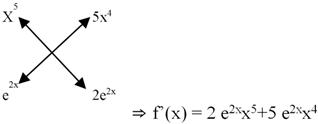2.6. Formula for 2nd Order: Repeat the Vertically and Crosswise in the 2nd Column Keeping First Column Fixed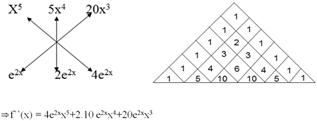2.7. Formula for 3rd Order: Repeating the Above Process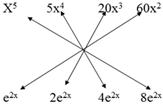f’’’ (x) = (8e2x)x5+3 (20 e2x) x4+3 (40e2x) x3+ (60 e2x)x2

Note: 1. Binomial expansion (Pascal’s Triangle) is used here.

Note: 2. The above process is equivalent to Leibnitz theorem for successive derivatives.

2.8. Special Cases for Integration By Parts

Formula 1: One mare than the one before,

∫x7dx = x8 + C, where C is the constant of integration.

Formula 2: Vertically successive derivative of algebraic function and successive integration of trigonometric function then used vertically and crosswise multiplication, the sign is adopted as +, -, + … alternately.

Example 1: ∫x2sin x

Now,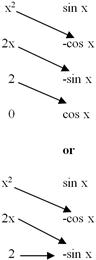In the 2nd way last term remains to be integrating.

∫x2sinx dx = - x2cosx + 2xsinx + 2 cosx + C, where C is the constant of integration.

Note: This can be done by the process of integration by parts. But the process expanding here is very short and easy. This process can be done until the derivative becomes zero.

Example 2: ∫x4sinx dx

Similarly proceed for the above expression as,

∫x4sinx dx= x4cosx +4x3sinx+12x2cosx - 24x3sinx - 24cosx+C, where C is the constant of integration.

Example 3: ∫x3log x dx (Algebraic and logarithmic functions)

Now,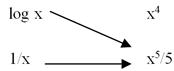∫x3log x dx = (x5) logx - ∫ (x5) dx = (x5) logx –x5dx + C, where C is the constant of integration.

Example 4: ∫ex sinx dx (Exponential and trigonometric functions)

I = ∫exsinx dx = - ex cosx + ex sinx +∫ex(- sinx) dx

= - excosx +ex sinx –I

(I/2)ex (- cosx+sinx)+C = ½ ex (sinx - cosx)+C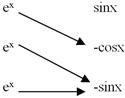Example 5: ∫eaxsinbx dx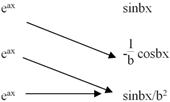I = (eax/b) cosbx + (a/b2)eaxsinbx – (a2/b2) ∫eaxsinbx dx

I= eax(a sinbx - bcosbx)/ 2 (a2+b2) + C 

3. Conclusions

Evidences show that before 100 years and now the contents are not significantly changed but algorithm and problem solving style is change. Also there was a very close way of thinking and value in mathematics. But later the major differences in their thinking of mathematics are only elaboration rather than the assimilation. But in algorithm and problem solving style the learner cannot assimilate for real understanding. Thus reformation of content, algorithm and process of problem solving need to be change with incorporating our classical mathematical developments.

I hope that there is beginning to see the paradigm shift that needs to happen. We need a major shift in societal expectation to include both language and mathematics in those preschool years. We need make mathematical culture for mathematics what they have always done for language and practical uses. That foundation in mathematics is every bit as important as it is for language. Our children need to be constantly reinforced and encouraged and praised for their persistence for mathematics as much as for language with evident the context in historical development. And maybe most important is that our children need a self concept that says "I am smart enough and I can learn this." For this we relate mathematics from early stage to now. They must never doubt their own ability to learn with relating to life and developmental facts. Hence in conclusion everyone in society needs to understand that they are an essential part of creating the foundation for a successful future in mathematics for our pre - school children by the linkage of classical to modern mathematics.

Acknowledgements

I express the deepest sense of gratitude and sincere thanks to the Editorial Board of Science Publishing Group for acceptance of my paper and suggestions. I extend my sincere thanks to my senior Professors; Shankar Raj Pant, Ram Man Shreshtha, Shantosh Man Maskey, Dinesh Raj Pant and Binod Dhakal for their continuous encouragements with suggestions and all sympathizers those who regularly supported the development of mathematics with preserving the classical mathematical developments.

References

1. Acharya, E. R. (2015), Naya Raj Pantaka Ganitiya Kritiharuko Adhyayan (An Unpublished PhD Dissertation): Dang, Nepal Sanskrit University, Nepal.
2. Bharati Krishna Tirthaji Maharaja (1992), Vedic Mathematics, Delhi: Motilal Banarsidass Publishers, Pvt. Ltd.
3. Casey, G. commentary of Lilavati. (Retrieved: 1Aug, 2014): http: //www.meritnation.com/ask - Answer/question.
4. Cooke, R. (1997). The History of Mathematics, New York, A Willey - Interscience Publication.
5. Dhananjaya, C. (1934). Shishubodha Tarangini, Ramghat, Vanaras: Gorakha Pustakalay.
6. Kenneth R. W. (1984), Discover Vedic mathematics, Delhi: Motilal Banarsidass Publishers.
7. Pande, G. (1883). Vyaktachandrika, Bombay: Nirnaya Sagar.
8. Pant, N.R. (1980). Gopal Pande & his rule of cube roots, Kathmandu: Nepal Academy.
9. Sudhakar, D. (n.d.). Commentary of Lilavati.
10. http: //www.powershow.com/view/7997a - ZTVhM/Bhaskara_II_powerpoint_ppt_presentation (Retrieved: 19 Sep., 2015).

 Contents 1. 2. 2.1. 2.2. 2.3. 2.4. 2.5. 2.6. 2.7. 2.8. 3.
Article ToolsAbstractPDF(5184K)